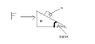# Dynamics: Moving wedge with mass on it

• bobthebanana

## Homework Statement

Small block of mass "m" rests on sloping side of triangular block of mass "M" which itself rests on table. Assuming all surfaces are frictionless, determine force "F" that must be applied to "M" so that "m" remains fixed. Wedge incline is "THETA"

## The Attempt at a Solution

attached is picture. I don't know where to start, so no attempted solution. The answer is:
F = (m+M)g*tan(THETA). i don't see why the mass on the wedge would move up the ramp if a force is applied to the left of the wedge.

where g is acceleration due to gravity

#### Attachments

•pic.GIF
907 bytes · Views: 536
Because of the pseudo force. If a pseudo force F is applied to the wedge, then the acceleration of the wedge+mass system is F/(M+m) and the force on m is mF/(M+m). The projection of that force along the surface of the wedge is equal to the gravitational force acting along the surface of the wedge.

k solved it thanks